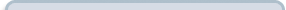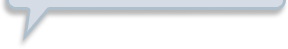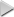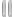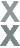# Fan Appreciation Night, Part 2

###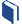Resources for this lesson: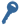Key Terms:

Intersection
Union

> Glossary> Calculator Resources> Teacher Resources: Instructional Notes## Create and Analyze

The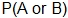is known as finding the probability of the intersection of A and B, and may also be written as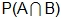.  Let’s explore what findingmeans for mutually inclusive events.  Since mutually inclusive events can occur at the same time, the situation can be represented with a Venn diagram: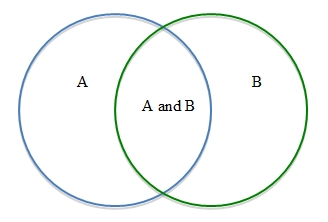To find the probability of the intersection of A or B,, we cannot simply find the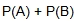, because the two probabilities overlap with the section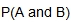.  This section would be counted twice.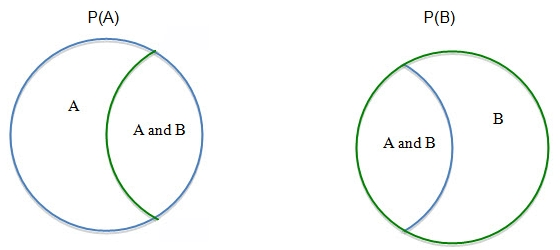Since the, the union of A and B, is counted twice, you would need to subtract one of these sections to find the.  So, when events are mutually inclusive, you apply the Addition Rule,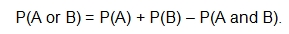Let’s explore how to find the probability of winning a t-shirt or a hat.